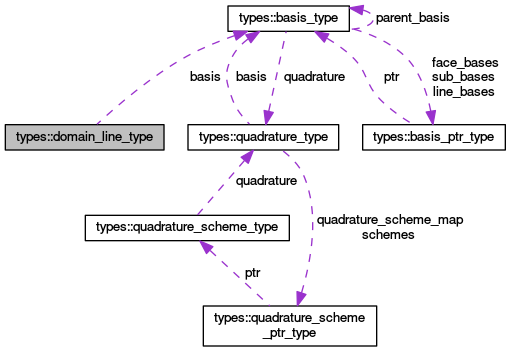OpenCMISS-Iron Internal API Documentation
types::domain_line_type Type Reference

Contains the information for a line in a domain. More...

Collaboration diagram for types::domain_line_type:[legend]

## Public Attributes

integer(intg) number
The line number in the domain. More...

type(basis_type), pointer basis
A pointer to the basis function for the line. More...

integer(intg), dimension(:), allocatable nodes_in_line
NODES_IN_LINE(nn). The local node number in the domain of the nn'th local node in the line. Old CMISS name NPL(2..5,nj,nl). More...

integer(intg), dimension(:,:,:), allocatable derivatives_in_line
DERIVATIVES_IN_LINE(i,local_derivative_idx,local_node_idx). When i=1 DERIVATIVES_IN_LINE will give the global derivative number of the local_derivative_idx'th local derivative for the local_node_idx'th local node in the line, When i=2 DERIVATIVES_IN_LINE will give the global derivative version number of the local_derivative_idx'th local derivative for the local_node_idx'th local node in the line. Old CMISS name NPL(4..5,nj,nl). More...

logical boundary_line
Is .TRUE. if the line is on the boundary of the mesh for the domain, .FALSE. if not. More...

integer(intg) element_number
The element number of the element on which the line is on. More...

## Detailed Description

Contains the information for a line in a domain.

Definition at line 622 of file types.f90.

## Member Data Documentation

 type(basis_type), pointer types::domain_line_type::basis

A pointer to the basis function for the line.

Definition at line 624 of file types.f90.

 logical types::domain_line_type::boundary_line

Is .TRUE. if the line is on the boundary of the mesh for the domain, .FALSE. if not.

Definition at line 627 of file types.f90.

 integer(intg), dimension(:,:,:), allocatable types::domain_line_type::derivatives_in_line

DERIVATIVES_IN_LINE(i,local_derivative_idx,local_node_idx). When i=1 DERIVATIVES_IN_LINE will give the global derivative number of the local_derivative_idx'th local derivative for the local_node_idx'th local node in the line, When i=2 DERIVATIVES_IN_LINE will give the global derivative version number of the local_derivative_idx'th local derivative for the local_node_idx'th local node in the line. Old CMISS name NPL(4..5,nj,nl).

Definition at line 626 of file types.f90.

 integer(intg) types::domain_line_type::element_number

The element number of the element on which the line is on.

Definition at line 628 of file types.f90.

 integer(intg), dimension(:), allocatable types::domain_line_type::nodes_in_line

NODES_IN_LINE(nn). The local node number in the domain of the nn'th local node in the line. Old CMISS name NPL(2..5,nj,nl).

Definition at line 625 of file types.f90.

 integer(intg) types::domain_line_type::number

The line number in the domain.

Definition at line 623 of file types.f90.